# Scared about Graph Data Structure, Understand in Lame Words 🙌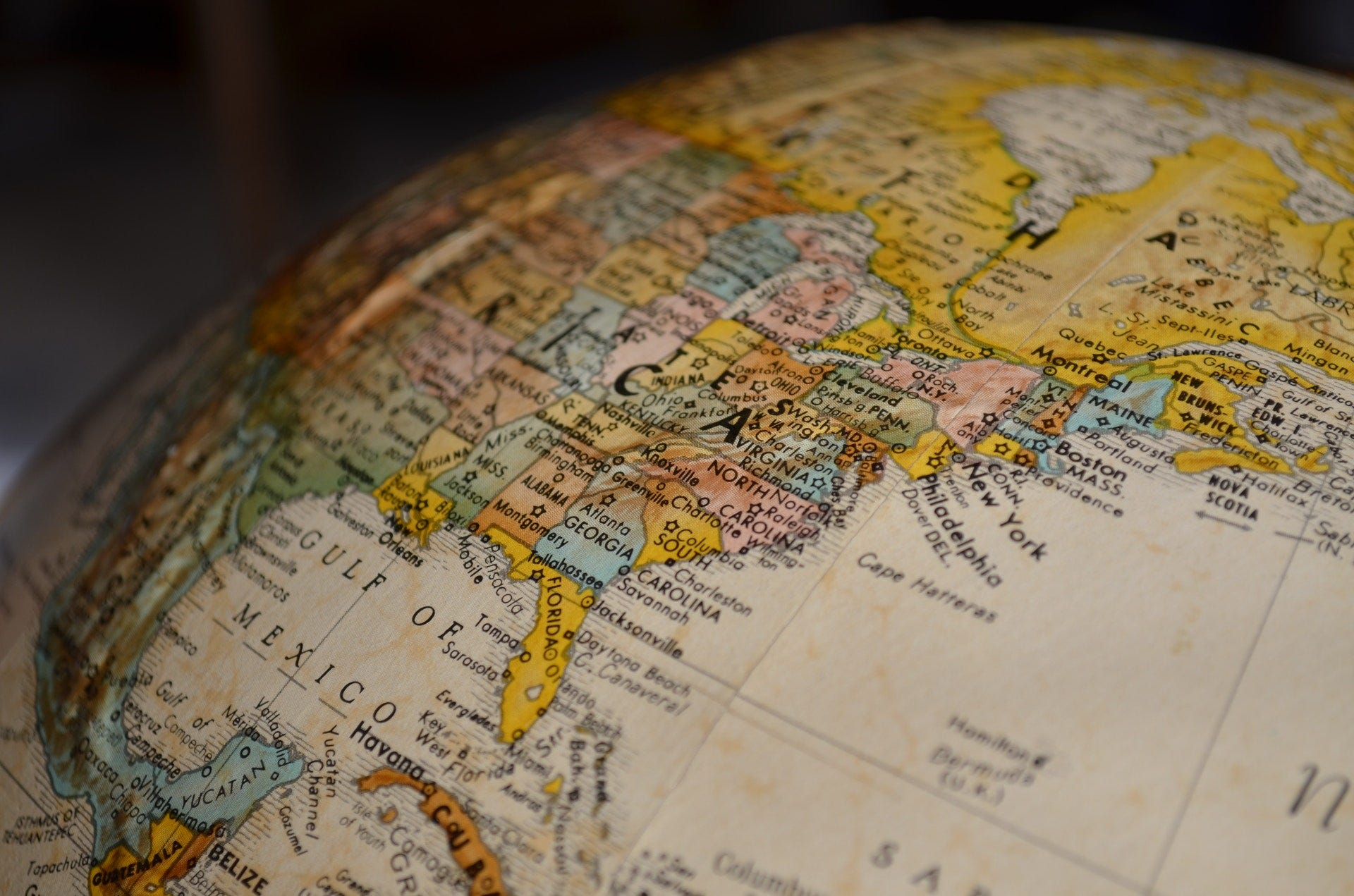Pexel image

Let's learn the most used data structure as in very simple language, graph is most useful and most used data structure in computer science when in come to modelling real life.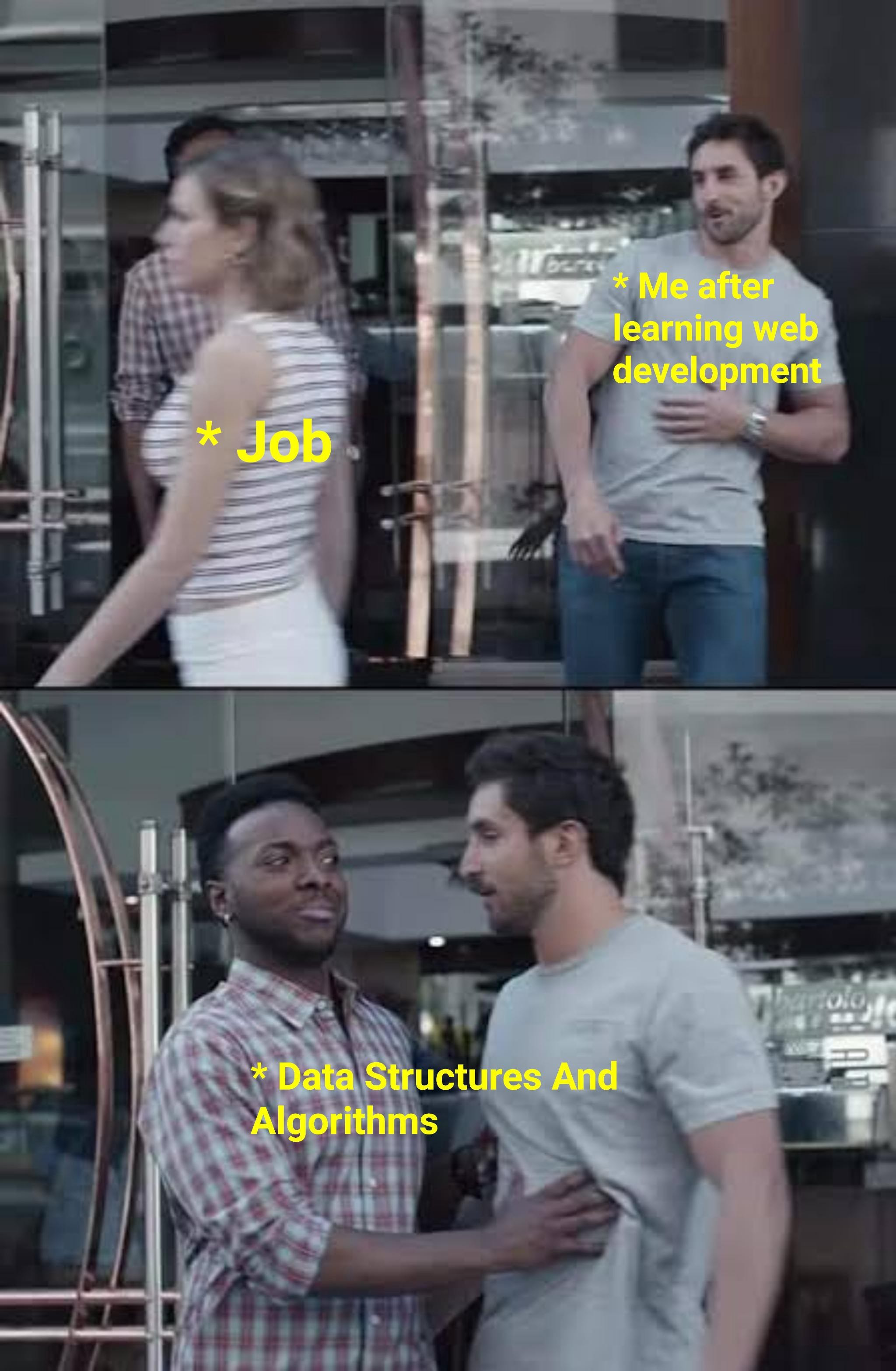DS meme

So let see what Wikipedia say about Graph -

In a computer science, a graph is an abstract data type that is meant to implement the undirected graph and directed graph concepts from the field of graph theory within a math.

Now understand in simple terms -

A graph is simply a set of value which are related in a pair vise fashion.

Even as today we can see disease like corona that's you can also see in graph. Corona Report Graph

As you can see in the above image it look like a network, a connection to a different node.

In a graph each item is called a node or a vertex, and then the node are connected with edges. We are lucky to have graph because graph are great data structure to model real world relationship like links which we are working with real thing that can define Graph more easily, the most common example we can come across like how internet works.

• We can represent a graph like friends list.
• Even check with family trees.
• Real life scenario like road (behave mostly to graphs) which connecting one city to another.
• Facebook uses for social networking, Amazon uses for recommendation engine and google recommended for Google map to show the shortest path between location.

If you have heard of linked list and tree, we can compare like this, all linked list are tree and all trees are graph.

Characteristic to describe graph

One thing you really want to give yourself familiar with the idea of —

• Directed graph
• Undirected graph

So these type of graph is describing the traffic flow. It some kind of system in which moment is bidirectional.

Undirected graph

Undirected graph one we can go any direction, for example let imagine undirected graph as a highway where you can go back and forth.

Example: Facebook, so facebook is undirected graph when we add anyone as friend, then you are connected to your friend and vice-versa.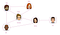Facebook example

Directed graph

Directed we can go to directed direction only.

Example:Twitter is more directed, if you follow anyone you can see all post of followed one, but followed one cannot see your post, so it kind of one direction flow.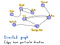Twitter example

Another way to describe graph

So, let see another way to describe the graph is -

• Weighted Graph
• Unweighted Graph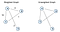Weighted and Unweighted graph

As you can see from image value can apply to various aspect of graph, as you can see values at the edge of the connection over graph, maybe we can find the more efficient way to visit sites.

Then you can try the weighted graph because every edge have a value.

Example of weighted graph

With the help of weighted graph google map can find the shortest path for you to get there. Whereas these type of graphs are most used to calculate the optimal path, like the fastest way to find the path from start to end.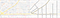Google map

Example of Unweighted graph

Social network (example Facebook) map where each vertex represents a person and the edge between them denotes if they are friends or not. Since relationship cannot be measured, like Ross and Joey are 50% friends or 60%friends, hence edges cannot be associated with any weight.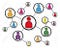Unweighted graph

Last but not the least final way to describe graph

• Cyclic
• Acyclic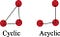Cyclic and Acyclic

Cyclic Graph

So, when you have a vertex connected to each other as a circular then its call a cycle, where you can go to one node to another and back to start.

Cyclic graph are common in weighted graph such as Google Map, where all node connected to each other and find a path

Acyclic Graph

In acyclic graph you have a vertex not connected or make a circle then its call an acyclic graph.

The Best example to understand the graph, one of my favorite Internet Graph. Where you can find the all internet in one graph, please explore the link and share your comment, how you feel about the internet graph.

Keep coding, thanks for reading this blog.

Read code • Learn Code • Write code • Relax • Write code, www.linkedin.com/in/ankit1srivastava

Read code • Learn Code • Write code • Relax • Write code, www.linkedin.com/in/ankit1srivastava